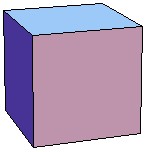index: click on a letter A B C D E F G H I J K L M N O P Q R S T U V W X Y Z A to Z index index: subject areas numbers & symbols sets, logic, proofs geometry algebra trigonometry advanced algebra & pre-calculus calculus advanced topics probability & statistics real world applications multimedia entrieswww.mathwords.com about mathwords website feedback

Cube
Regular Hexahedron

A regular polyhedron for which all faces are squares.

Note: It is one of the five platonic solids.

 Cube a = length of an edge Volume = a3 Surface Area = 6a2Rotate me if your browser is Java-enabled.